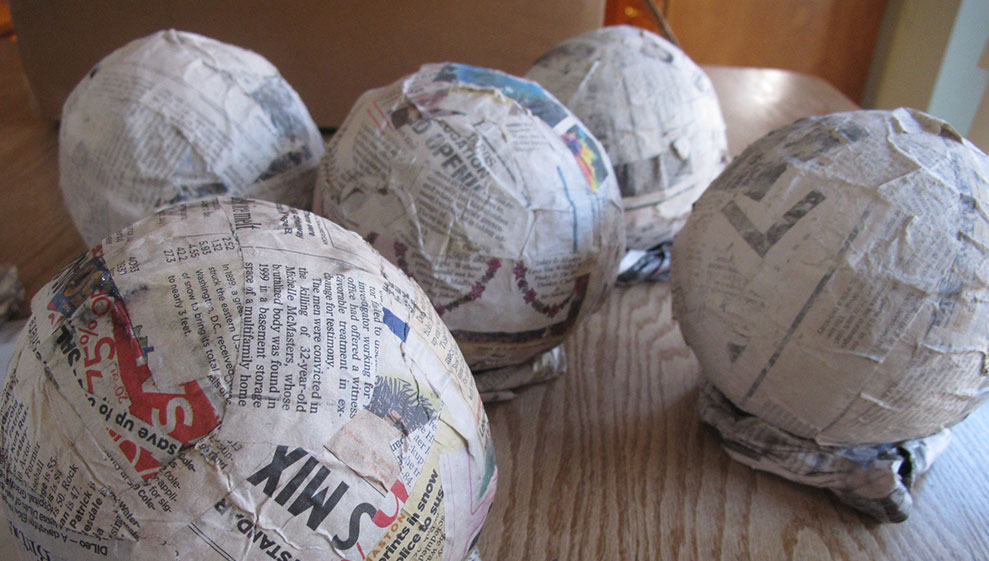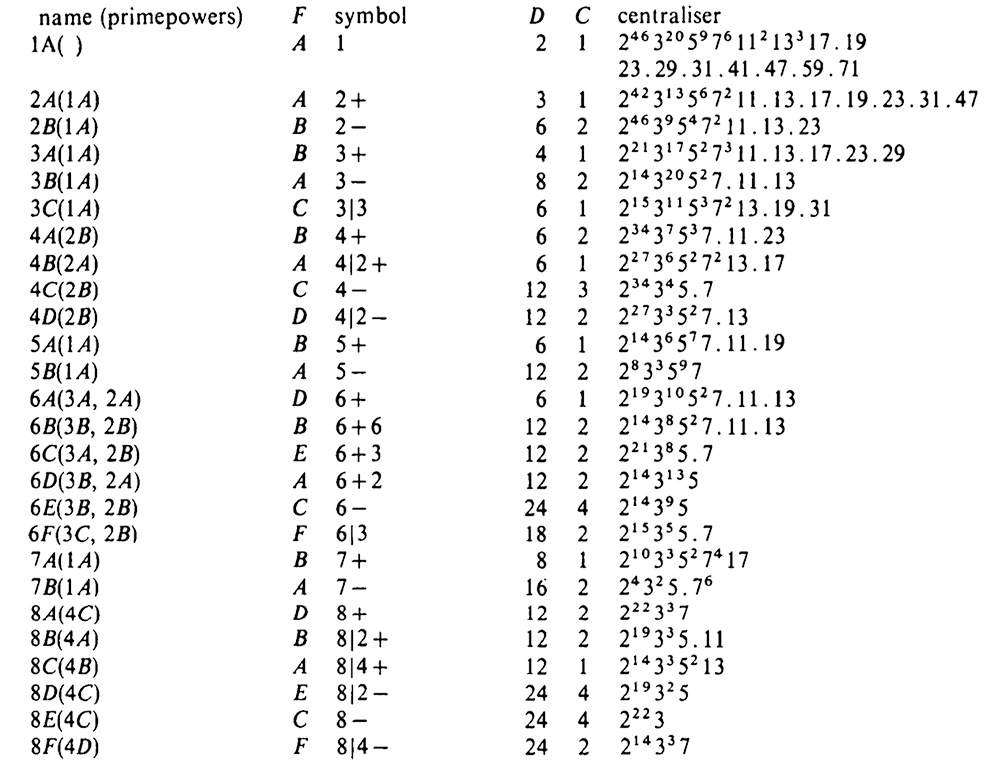# Tag: Sebbar

One of the better opening quotes of a paper:

“Even quite ungainly objects, like chairs and tables, will become almost spherical if you wrap them in enough newspaper.”The paper in question is The orbifold notation for surface groups by John Conway.

Here’s Conway talking leisurely about Thurston’s idea to capture the acting group via the topology of the orbifold space and his own notation for such orbifolds.

Here’s another version of the paper, with illustrations: The orbifold notation for two-dimensional groups, by Conway and Daniel H. Huson.

A very accessible account are these lecture notes:

A field guide to the orbifolds, notes from class on “Geometry and the Imagination” in Minneapolis, with John Conway, Peter Doyle, Jane Gilman and Bill Thurston, on June 17–28, 1991.

And, here are notes by Thurston on The Geometry and Topology of Three-Manifolds, including stuff about orbifolds.

I came across these papers struggling my way through On the discrete groups of moonshine by Conway, McKay and Sebbar.

On the genus $0$ property of moonshine groups they have this to say:

“As for groups of the form $(n|h)+e,f,\dots$, the genus can be determined from the fundamental regions using the Riemann-Hurwitz formula. Since most of the groups are not subgroups of the modular group, the calculations of the genus, which cannot be produced here because of their length, are carried out by finding the elliptic fixed points and the cone points in the orbifolds attached to the fundamental regions. The Euler characteristic of the orbifold determines the genus of the group. See [paper] for more details on orbifold techniques.”

Monstrous moonshine associates to every element of order $n$ of the monster group $\mathbb{M}$ an arithmetic group of the form
$(n|h)+e,f,\dots$
where $h$ is a divisor of $24$ and of $n$ and where $e,f,\dots$ are divisors of $\frac{n}{h}$ coprime with its quotient.

In snakes, spines, and all that we’ve constructed the arithmetic group
$\Gamma_0(n|h)+e,f,\dots$
which normalizes $\Gamma_0(N)$ for $N=h.n$. If $h=1$ then this group is the moonshine group $(n|h)+e,f,\dots$, but for $h > 1$ the moonshine group is a specific subgroup of index $h$ in $\Gamma_0(n|h)+e,f,\dots$.

I’m sure one can describe this subgroup explicitly in each case by analysing the action of the finite group $(\Gamma_0(n|h)+e,f,\dots)/\Gamma_0(N)$ on the $(N|1)$-snake. Some examples were worked out by John Duncan in his paper Arithmetic groups and the affine E8 Dynkin diagram.

But at the moment I don’t understand the general construction given by Conway, McKay and Sebbar in On the discrete groups of moonshine. I’m stuck at the last sentence of (2) in section 3. Nothing a copy of Charles Ferenbaugh Ph. D. thesis cannot fix.

The correspondence between the conjugacy classes of the Monster and these arithmetic groups takes up 3 pages in Conway & Norton’s Monstrous Moonshine. Here’s the beginning of it.